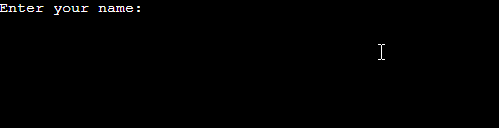# Check if user input is empty in python

## 📋 Table Of Content

In this article, we will learn to detect if the user input is empty or not in python.

In Python, we can take user input using the `input` function. The input function returns the user input data as a string.

To detect if the user input is an empty string, we can use the `if` statement to compare the user input with an empty string, eg `user_input == ''`.

## Check if user input is empty using if statement

Here, we will use the `if` statement and compare the user input value to an empty string.

Example:

``````user_input = input('Enter your name: ')

if user_input == '':
print('The input is Empty')
``````

Output:

``````Enter your name:
The input is Empty
``````

Since the `input()` function returns a string, we can use the equality operator (`==`) to compare the input with an empty string.

If the `if` condition returns `True`, it will print out "The input is Empty" in the terminal.

However, if we just enter a space or whitespace, it will not be considered empty because even though it looks empty, it is a non-zero length character.

So to fix it, we use the `strip` method to remove all the leading and trailing whitespaces from the input value and then compare it with an empty string.

``````user_input = input('Enter your name: ')

if user_input.strip() == '':
print('The input is Empty')
``````

Now, if we want to prevent a user to enter an empty string we can use the while loop.

## Prevent user from entering empty string using while loop

A `while` loop executes a set of statements as long as the condition is `True`. So we can prompt the user to input their name until they enter a non-empty string.

Example:

``````while True:
user_input = input('Enter your name: ')

if user_input.strip() != '':
print(f'Thanks {user_input} ')
break
``````

The above code will keep on prompting the user to enter the name until they enter their name.

Output:And once the user inputs a valid string the `break` statement will execute and it will break out (exit) of the while loop.

## Check if user input is empty using the not operator

The `not` is a logical operator in python, which returns `True` if the statement is false and `False` if the statement is true. It just reverses the boolean of the statement.

Using this operator with the `if else` statement we can detect if the user input is empty or not.

Example:

``````user_input = input('Enter your name: ')

if not user_input.strip():
print('The input is Empty')
else:
print(f'thanks {user_input}')
``````

Output:

``````#empty string
Here, when the user enters an empty string the first block turn `True` and we get "The input is empty " in our terminal. And if the input is non-empty, the else statement is executed.